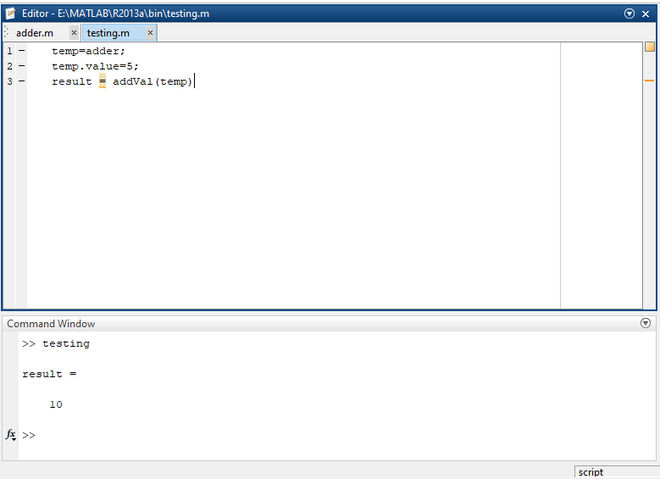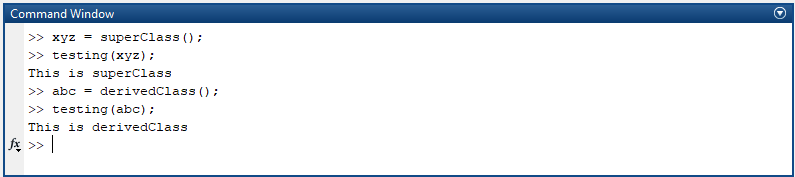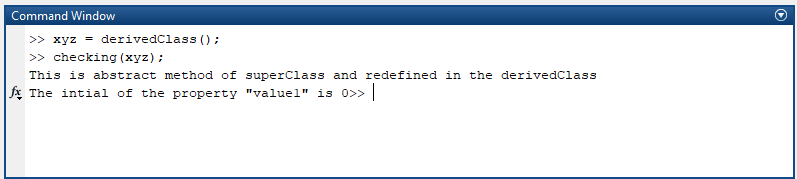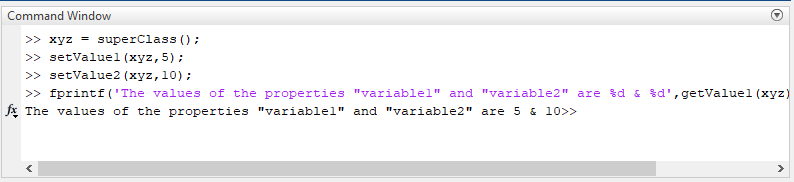# Object Oriented Programming (OOPs) in MATLAB

• Difficulty Level : Hard
• Last Updated : 17 Aug, 2021

Object-Oriented Programming (OOPs) in MATLAB is similar to many conventional programming languages like Java, Python, etc except the syntax. The important feature of OOPs is, it enables you to combine data and it’s associated actions (methods/functions) into objects. Compared to other languages, such as C++, Java, etc, Object-Oriented Programming will be much faster in MATLAB which enables you to solve complex computing applications. There are certain features in OOPs that can be used for solving complex problems, providing security to the data, etc.

OOPs features supported by MATLAB are:

• Object
• Class
• Polymorphism
• Inheritance
• Abstraction
• Encapsulation

Now let’s dive into some examples to understand the above concepts better.

## Class

A Class is a template/blueprint defined by a user using which objects are created.  It comprises of a set of attributes and methods which are used for object entities. A Constructor is a method/function, defined with the same name of the class, and it initializes the classes with certain values passed through the calling function (i.e Constructor). Defining a constructor is optional as in many other languages. MATLAB syntax is quite peculiar compared to other conventional programming languages.

Syntax:

classdef (attributes) className

. . . . .

end

Parameters:

classdef       ->   keyword used to define classes

attributes     ->    Arguments/Values used to modify the behaviour of class

Example:

## Matlab

 `% MATLAB program to illustrate Classes`   `classdef` `adder` `    `  `    ``% Attributes of a class are defined` `    ``% in properties block` `    ``properties` `        ``value` `    ``end` `    `  `    ``% Function/Methods of class are defined` `    ``% in methods block` `    ``methods` `        ``function` `res = addVal(obj)` `            ``res = obj.value+obj.value;` `        ``end` `    ``end` `end`

Save the above code as adder.m file and access it with commands from command prompt/another matlab file. Let’s access the above code by giving some commands from another matlab file and observe the output to understand it better.

## Matlab

 `% MATLAB program to access the` `% user defined classes`   `% Initiating the Class` `temp=testing();`   `% Assign values to the class attributes` `temp.value=5;`   `% Calling methods inside the class` `addVal(temp)`

Save the above code as testing.m. After executing the above file, you will get the following output.Output

### Object

An Object is a logical entity that interacts with the user-defined class by invoking methods/functions. We can create as many objects as you want. Every object will have two characteristics i.e., state and behavior. These characteristics vary with every object as per the situation. For example, dog is an object/identity. It’s loyalty, sleep, walk, etc are behaviors and size, color, breed, etc comes under the state.

### Inheritance

The title itself suggests that inheritance is nothing but inheriting/acquiring all the properties, such as attributes, methods, etc, of parent/base class. The class that is derived from a base class is called as Sub Class/Child Class and it inherits all the properties of its parents class. Superclass is the class that is being inherited by the subclass. The importance of the inheritance is the code reusability such as using the parent class’s attributes, methods, etc. Let’s dive into an example to understand the inheritance concept better.

Syntax:

classdef subClassName < SuperClass

. . . . . . .

end

Super Class:

## Matlab

 `% MATLAB program to illustrate Super class` `% and inheritance.`   `classdef` `Animal` `    `  `    ``methods`   `        ``function` `eat(self)` `            ``disp(``'I am Eating'``);` `        ``end`   `        ``function` `sleep(self)` `            ``disp(``'I am Sleeping'``);` `        ``end` `       `  `    ``end` `    `  `end`

Save the above code as Animal.m and this is our superclass. Let us see subclass now.

## Matlab

 `% MATLAB program to illustrate` `% sub class and inheritance`   `classdef` `Lion < Animal` `    `  `    ``methods` `        `  `        ``function` `roar(self)` `            ``disp(``'Roaring'``);` `        ``end` `        `  `    ``end` `    `  `end`

Save the above code as Lion.m and this is our subclass. Now let’s access the parent class using subclass object and observe the output.

Output:Output

### Polymorphism

The mechanism of defining a function with the name of the already existed function is known as polymorphism. Simply we call it as overriding. MATLAB doesn’t support overloading a function. The polymorphic functions perform different operations with the same name based on the passed arguments and user-defined logic.

SuperClass:

## Matlab

 `% An example of polymorphism in MATLAB`   `classdef` `superClass` `   `  `    ``methods` `    `  `        ``function` `testing(self)` `            ``disp(``'This is superClass'``);` `        ``end` `        `  `    ``end` `    `  `end`

Save the above code as superClass.m and let’s also see the derived class code.

Derived Class:

## Matlab

 `% An example of polymorphism in MATLAB`   `classdef` `derivedClass < superClass` `    `  `    ``methods` `    `  `        ``% Overriding the superClass method` `        ``function` `testing(self)` `            ``disp(``'This is derivedClass'``);` `        ``end` `    ``end` `    `  `end`

Save the above code as derivedClass.m and let’s observe the output.

Output:Output

### Abstraction

An Abstract class depicts the functionality performed by a group of classes. It hides important or unnecessary data and shows only the essential components. For instance, A mobile can be viewed but not its inner components. All the methods, attributes that are being used by the subclasses are declared in the abstract class. A concrete subclass is derived from abstract class to use its properties. An abstract can’t be instantiated. It can only be inherited. All the methods declared in the abstract class must be redefined/overridden in the subclass.

Syntax:

classdef (Abstract) className:

. . . . .

end

Example:

## Matlab

 `% An example of abstract class in MATLAB`   `classdef` `(Abstract) superClass` `    `  `    ``% Declaring abstract properties` `    ``properties (Abstract)` `        ``value1` `    ``end` `    `  `    ``% Declaring abstract methods` `    ``methods (Abstract)` `        ``checking(obj)` `    ``end` `    `  `end`

Save the above code as superClass.m and let’s see the code for the base class.

## Matlab

 `% An example of abstract base class in MATLAB`   `classdef` `derivedClass < superClass` `    `  `    ``% Redefining abstract properties` `    ``properties` `        ``value1 = 0;` `    ``end` `    `  `    ``% Redefining abstract methods` `    ``methods` `        ``function` `checking(self)` `            ``disp(``'This is abstract method of superClass and redefined in the derivedClass'``);` `            ``fprintf(``'The initial of the property "value1" is %d'``,self.value1);` `        ``end` `    ``end` `    `  `end`

Save the above code as derivedClass.m and execute the code and observe the output by giving a couple of commands.

Output:Output

### Encapsulation

Prerequisite: MATLAB documentation of Value and Handle Classes

Encapsulation is a mechanism of enclosing the data and the code together in a single class/unit. It provides security to the data by prohibiting the limit to other classes. One can access or modify the data of a class only by using getters and setters methods. We need to inherit handle class to implement encapsulation in MATLAB as it doesn’t return duplicate and modified objects and also handles the errors.

MATLAB –

Save the above code as superClass.m and let’s observe the output by giving a couple of commands.

Output:Output

Note:

• In MATLAB, Class variables are called as Properties.
• In MATLAB, Classes and methods are defined in separate files.
• We have to save the class files and method files with their respective names.

My Personal Notes arrow_drop_up
Recommended Articles
Page :